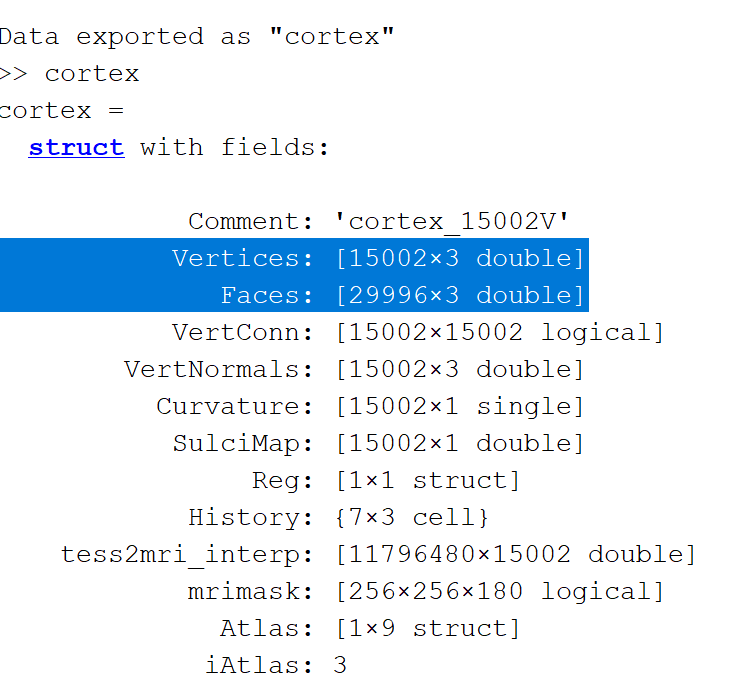# Transforming segmented brain into FEM mesh

Hi,
after having computed my head model (with CAT12), I obtain the following head model (see image) with 2002 vertices. To compute the Gain Matrix I use a 3-shell sphere model.
I obtain a matrix having 3x2002 columns. So I suppose that a lead field function has been computed for each vertice of my model. Is this correct ?

Now I would like to export this head model to plot it as a FEM mesh, using paraview (I'm writing my own routine to obtain a .vtk). To that I have the coordinates of the vertices (which will become nodes), but I also need the connectivity matrix to define the tetrahedral elements.
How can I have this information ? My goal is to obtain exactly the same model that the one the attached image.
Thanks for your help.
3D_Subject01.tif (194.5 KB)
Greg

Hi,

Yes, you have computed a lead field using the 3shell method from the source space formed by the cortex (2002 nodes).

It seems that there is nothing related to the FEM in your computation, you are using a set of nodes that forms a cortical surface and you have used the 3shell method to compute the lead field (analytical solution).

In the attached image, you have the cortex with 2002 vertices, and it's a surface mesh (BEM) and note a volume mesh (FEM). You can export this surface to matlab ==> right click on the cortex, ==> file ==> export to matlab ==> give any variable name

then you will have the list of the vertices (nodes) and also the list of the faces (connectivity), but they are triangles and not tetrahedron.From here you can export the mesh using you own functions, but it's a surface mesh and not a volume.
If you want to have FEM mesh, you can refer to these tutos :
https://neuroimage.usc.edu/brainstorm/Tutorials/Duneuro